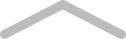# Introduction to CFDs

In this CFD tutorial, we will present an illustration of a CFD trading case study to show you how CFD trading works and help you have a very clear idea about how this works. Lets say we have a cash float in our hand that is worth of \$8000 and the CFD broker that we are working with is offering a 10:1 leverage. This means, our float is leveraged up to \$80,000.

Also lets assume that we will use a fixed trade size of \$8,000 per trade for our trading system. So this way we will be able to enter as many as 10 positions at a time by using the float we have in hand. However, you need to keep this in mind that the numbers that we have used here are only for illustration. This particular money management may not be suitable for your trading system. To figure out your version of money management rules, you need to do some backtesting.

## CFD Trading Mechanics: The Calculations

Now, lets assume that you bought CFDs when the price was \$5.50. Since the trade size that we are working with is \$8000, the number of CFDs that was bought would be 8000/5.50 = 1454.

Note: this particular trade size is completely hypothetical and is neither a suggestion of how to perform money management, nor a part of any recommended system. This is solely an example to make you understand the basic process.

Let's continue with this CFD tutorial. Now let’s assume that the stop loss was set at \$5.25, means that if the price falls to or below the \$5.25 mark, we will exit this trade at a loss. Assuming that the trade goes well and that the CFD price of the CFD is now \$5.75 and we have trailed the stop up to \$5.40. A trailing stop refers to the stop that shifts in the direction of our trade (up for long positions) when the trade goes in our direction. Now if the price goes up to \$6.00 and our trailing stop is moved to \$5.90 then when the price of the CFD will fall, our stop loss will exit us from the trade at \$5.90. The whole trade took about 12 days.

So, the difference between the entry and exit price is: \$5.90 - \$5.50 = \$0.40

From this, we can calculate our gross profit = (difference between entry and exit price) x (number of CFDs) = 1454 x 0.40 = \$581

Now that we have our gross profit, it is time to calculate the costs to find out the net profit.
Cost = commission + interest
First we will calculate the commission and then interest.

1. Assuming that we will have to pay \$15 in and \$15 out or 0.15% of the size of the trade. The one that is greater is needed to be taken under consideration. Here we will take the first one since this is greater. So, the commission would be \$15 + \$15 = \$30
2. Also, assume that the interest rate charge for the provider for long positions held overnight is 7% or 0.07 per annum. In order to find out how much this is in case of our trade, we need to make it "pro rata" (times it by the days in trade, then divide by 365), and then will have to multiply it by the size of the trade.

Interest = (interest rate for long position) x (days in trade/365) x (trade size) = 0.07 x 12/365 x 8000 = \$18.41

So our net profit is going to be: Gross Profit – (commission + interest) = \$581 – (30 + 18.41) = \$532.59

So, here goes your first CFD trade with all the calculations. Note that the trade that we have described above is completely hypothetical and not taken from any particular system.

You need to keep in mind that in case of short positions, interest costs are paid to you, not charged, so this will offset the costs rather than contribute in it. The actual figure for interest payment is usually a bit more complex than the way we have illustrated it in this example due to the fact that the interest rate is not calculated from the value of the trade size during the entering or exiting the position but at a daily basis from the value of the trade size at market value. For example, if we consider the interest cost based on our final trading size which is 8532.59, the interest would be \$19.63. So you can see there is not much of difference in between. So we can say that the actual interest cost would be within the range of \$18 and \$19. This is how the CFD trading is executed. Here you have learnt how the leverage works along with the calculation of the costs that are involved in CFD trading.Log in or Join Tablo to comment on this chapter...
~
69 mins to go